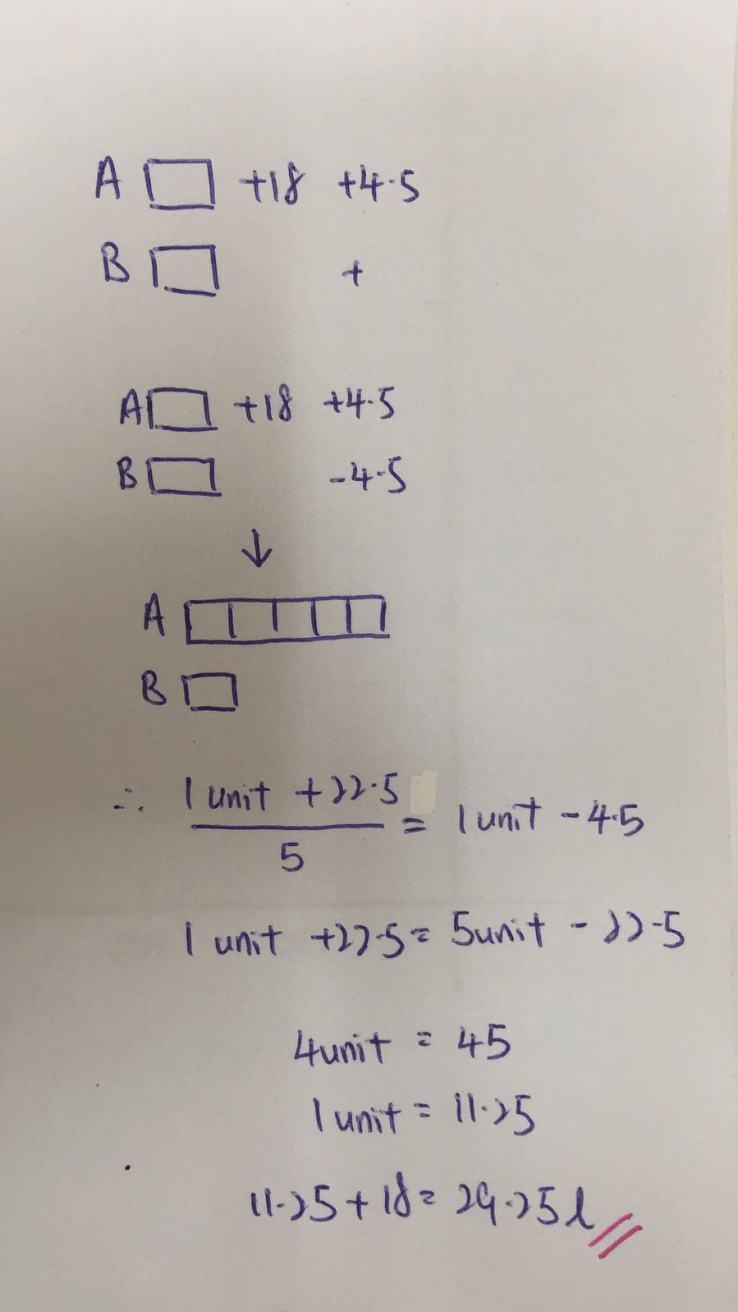# Question

Need help for below question:

Tank A holds 18.l of petrol more than Tank B.

After transferring 4.5l of petrol from Tank B to Tank A, Tank B had 1/5 as much as Tank A.

How much petrol was in Tank A at first?

Source: South View Primary0 Replies 0 Likes

At first —
Tank A : 1 unit + 18
Tank B : 1 unit

After —
Tank A : 1 unit + 18 + 4.5 = 1 unit + 22.5
Tank B : 1 unit – 4.5

1 unit + 22.5 = (1 unit – 4.5) x 5 = 5 units – 22.5
4 units = 22.5 + 22.5 = 45
1 unit = 45/4 = 11.25
1 unit + 18 = 11.25 + 18 = 29.25

Ans : 29.25 l.

0 Replies 0 Likes